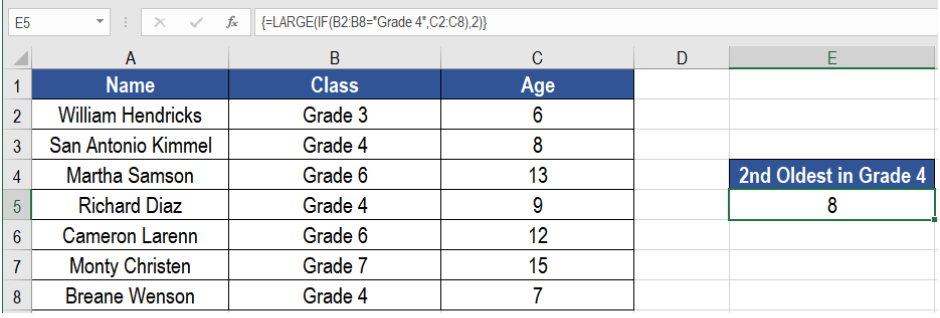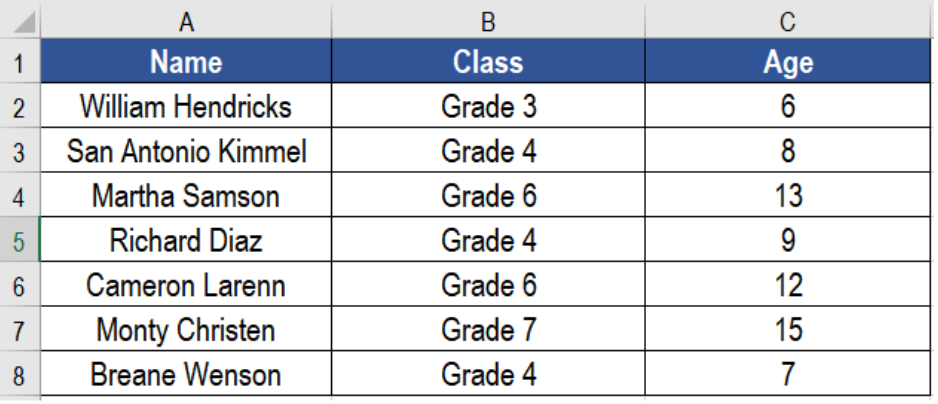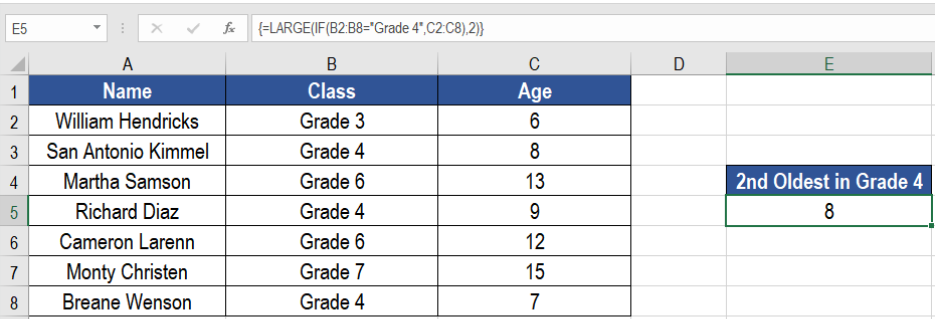Get instant live expert help with Excel or Google Sheets“My Excelchat expert helped me in less than 20 minutes, saving me what would have been 5 hours of work!”

#### Post your problem and you'll get expert help in seconds

Your message must be at least 40 characters
Our professional experts are available now. Your privacy is guaranteed.

# Large with criteria

Analyzing data often requires to find the largest value. Sometimes we need to extract the largest value based on a criteria. Excel offers a great solution to find the largest value with criteria. The LARGE and IF functions help us to find the largest value with criteria. In this tutorial, we will learn how to find the largest value with criteria in Excel.Figure 1. Example of How to Use Large with Criteria in Excel

## Generic Formula

`{=LARGE(IF(criteria,values),n)}`

Here, we use the functions LARGE and IF. LARGE retrieves the “nth” largest value from a numeric data. The IF function here performs a logical test on the range to see if they fulfil the criteria. The values that satisfy the criteria returns those values. The ones that does not satisfy the condition returns FALSE. This array is returned inside the LARGE function. We hardcode the number k for the kth value. This returns the kth largest number.

## Setting up Data

The following example uses a student information database. Column A, B and C has the names, ids and agesFigure 2. The Sample Data

To find out the second highest age in grade 4, we need to

• Go to cell E5.
• Assign the formula `=LARGE(IF(B2:B8=”Grade 4”,C2:C8),2)` to E5.
• Press Ctrl + Shift + Enter to apply it as an array formula.Figure 3. Applying the Formula

This will show the second highest age, which is 8.

## Notes

We can find the largest value based on multiple criteria as well. To do that we need to extend the formula based on boolean logic. The formula would have the syntax `{=LARGE(IF((criteria 1)*(criteria 2),value),n)}`. This will find the nth largest value based on multiple criteria.

Most of the time, the problem you will need to solve will be more complex than a simple application of a formula or function. If you want to save hours of research and frustration, try our live Excelchat service! Our Excel Experts are available 24/7 to answer any Excel question you may have. We guarantee a connection within 30 seconds and a customized solution within 20 minutes.

### Did this post not answer your question? Get a solution from connecting with the expert.Another blog reader asked this question today on Excelchat:## Subscribe to Excelchat.coAnother blog reader asked this question today on Excelchat: